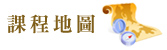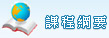回上一層
 微積分(含實習1H)(ECON104) 課程概要 中文概要 本課程授課內容分別陳述如下：(1)數學基本觀念。(2)導函數。(3)微分方法。(4)微分應用。(5)積分原理：黎曼和。(6)微積分基本定理。(7)積分方法。(8)偏微分。(9)受限制條件的極大化(Lagrange乘數的運用)。(10)簡單經濟應用。(11)重積分。(12)簡單數列級數和泰勒展開式。 英文概要 The contents include the following parts: (1) functions, limits, and derivative, (2) basic rules of differentiation. (3) applications of the derivative, (4) exponential and logarithmic functions, (5) definite integral and Riemann sum, (6) the fundamental theorem of calculus, (7) applications of the definite integral, (8) partial derivatives, (9) constrained maxima and minima and the method of Lagrange multipliers, (10) total differentials and double integrals, and (11) Taylor polynomials and infinite series. ※為加強推動保護智慧財產權觀念，請勿於合理使用範圍外，非法影印或重製書籍以免觸法。